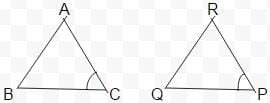Test: Congruence of Triangles

# Test: Congruence of Triangles

Test Description

## 10 Questions MCQ Test Mathematics (Maths) Class 7 | Test: Congruence of Triangles

Test: Congruence of Triangles for Class 7 2022 is part of Mathematics (Maths) Class 7 preparation. The Test: Congruence of Triangles questions and answers have been prepared according to the Class 7 exam syllabus.The Test: Congruence of Triangles MCQs are made for Class 7 2022 Exam. Find important definitions, questions, notes, meanings, examples, exercises, MCQs and online tests for Test: Congruence of Triangles below.
Solutions of Test: Congruence of Triangles questions in English are available as part of our Mathematics (Maths) Class 7 for Class 7 & Test: Congruence of Triangles solutions in Hindi for Mathematics (Maths) Class 7 course. Download more important topics, notes, lectures and mock test series for Class 7 Exam by signing up for free. Attempt Test: Congruence of Triangles | 10 questions in 10 minutes | Mock test for Class 7 preparation | Free important questions MCQ to study Mathematics (Maths) Class 7 for Class 7 Exam | Download free PDF with solutions
 1 Crore+ students have signed up on EduRev. Have you?
Test: Congruence of Triangles - Question 1

### Two angles are congruent if they have

Detailed Solution for Test: Congruence of Triangles - Question 1

When 2 angles are equal in measurement, they are said to be congruent.

Test: Congruence of Triangles - Question 2

### Which of the following is not a congruence criterion?

Detailed Solution for Test: Congruence of Triangles - Question 2

We have ASA ,SSS,SAS and RHS are congruence criterions.

Test: Congruence of Triangles - Question 3

### Which angle is included between the sides DE and EF of △DEF?

Detailed Solution for Test: Congruence of Triangles - Question 3

When you join the line DE and EF you will find a common vertex E , which is the included angle.

Test: Congruence of Triangles - Question 4

In ΔABC and ΔPQR, AB = 4 cm, BC = 5 cm, AC = 6 cm and PQ = 4 cm. QR = 5 cm. PR = 6 cm. then which of the following is true?

Detailed Solution for Test: Congruence of Triangles - Question 4

In the triangles we have AB=PQ,BC=QR and AC=PR , so we write the name of the triangles such that the corresponding sides are equal, ie, AB=PQ so it will beABC ≅ PQR

Test: Congruence of Triangles - Question 5

If ΔDEF ≅ ΔBCA, then the part of ΔBCA that correspond to ∠E is

Detailed Solution for Test: Congruence of Triangles - Question 5

In a congruence , triangles’ name are written such that the corresponding angles of both the triangles are equal, meaning that ∠D=∠B,∠E=∠C and ∠F=∠A. So , ∠C is the correct answer.

Test: Congruence of Triangles - Question 6

If ΔDEF ≅ ΔACB, then the part of ΔACB that correspond to ∠F is

Detailed Solution for Test: Congruence of Triangles - Question 6

In a congruence , triangles’ name are written such that the corresponding angles of both the triangles are equal, meaning that ∠D=∠A,∠E=∠C and ∠F=∠B. So , ∠B is the correct answer.

Test: Congruence of Triangles - Question 7

ΔABC and ΔPQR are congruent under the correspondence: ABC ↔ RQP, then the part of ΔABC that correspond to ∠P is

Detailed Solution for Test: Congruence of Triangles - Question 7From above diagram, it is clear that ∠C corresponds to ∠P

Test: Congruence of Triangles - Question 8

ΔABC and ΔPQR are congruent under the correspondence: ABC ↔ RQP, then the part of ΔABC that correspond to ∠Q is

Test: Congruence of Triangles - Question 9

ΔABC and ΔPQR are congruent under the correspondence: ABC ↔ RPQ, then the part of ΔABC that correspond to PQ is

Test: Congruence of Triangles - Question 10

What is the side included between the angles A and C of △ABC?

## Mathematics (Maths) Class 7

168 videos|252 docs|45 tests
 Use Code STAYHOME200 and get INR 200 additional OFF Use Coupon Code
Information about Test: Congruence of Triangles Page
In this test you can find the Exam questions for Test: Congruence of Triangles solved & explained in the simplest way possible. Besides giving Questions and answers for Test: Congruence of Triangles, EduRev gives you an ample number of Online tests for practice

## Mathematics (Maths) Class 7

168 videos|252 docs|45 tests